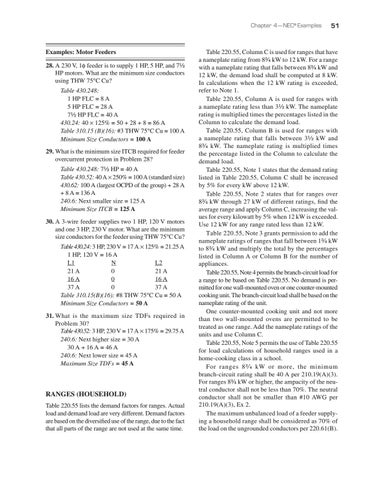Chapter 4 — NEC® Examples

Examples: Motor Feeders 28. A 230 V, 1φ feeder is to supply 1 HP, 5 HP, and 7¹⁄₂ HP motors. What are the minimum size conductors using THW 75°C Cu? Table 430.248: 1 HP FLC = 8 A 5 HP FLC = 28 A 7¹⁄₂ HP FLC = 40 A 430.24: 40 × 125% = 50 + 28 + 8 = 86 A Table 310.15 (B)(16): #3 THW 75°C Cu = 100 A Minimum Size Conductors = 100 A 29. What is the minimum size ITCB required for feeder overcurrent protection in Problem 28? Table 430.248: 7¹⁄₂ HP = 40 A Table 430.52: 40 A × 250% = 100 A (standard size) 430.62: 100 A (largest OCPD of the group) + 28 A + 8 A = 136 A 240.6: Next smaller size = 125 A Minimum Size ITCB = 125 A 30. A 3-wire feeder supplies two 1 HP, 120 V motors and one 3 HP, 230 V motor. What are the minimum size conductors for the feeder using THW 75°C Cu? Table 430.24: 3 HP, 230 V = 17 A × 125% = 21.25 A 1 HP, 120 V = 16 A L1 N L2 21 A 0 21 A 16 A 0 16 A 37 A 0 37 A Table 310.15(B)(16): #8 THW 75°C Cu = 50 A Minimum Size Conductors = 50 A 31. What is the maximum size TDFs required in Problem 30? Table 430.52: 3 HP, 230 V = 17 A × 175% = 29.75 A 240.6: Next higher size = 30 A 30 A + 16 A = 46 A 240.6: Next lower size = 45 A Maximum Size TDFs = 45 A

RANGES (HOUSEHOLD) Table 220.55 lists the demand factors for ranges. Actual load and demand load are very different. Demand factors are based on the diversified use of the range, due to the fact that all parts of the range are not used at the same time.

51

Table 220.55, Column C is used for ranges that have a nameplate rating from 8³⁄₄ kW to 12 kW. For a range with a nameplate rating that falls between 8³⁄₄ kW and 12 kW, the demand load shall be computed at 8 kW. In calculations when the 12 kW rating is exceeded, refer to Note 1. Table 220.55, Column A is used for ranges with a nameplate rating less than 3¹⁄₂ kW. The nameplate rating is multiplied times the percentages listed in the Column to calculate the demand load. Table 220.55, Column B is used for ranges with a nameplate rating that falls between 3¹⁄₂ kW and 8³⁄₄ kW. The nameplate rating is multiplied times the percentage listed in the Column to calculate the demand load. Table 220.55, Note 1 states that the demand rating listed in Table 220.55, Column C shall be increased by 5% for every kW above 12 kW. Table 220.55, Note 2 states that for ranges over 8³⁄₄ kW through 27 kW of different ratings, find the average range and apply Column C, increasing the values for every kilowatt by 5% when 12 kW is exceeded. Use 12 kW for any range rated less than 12 kW. Table 220.55, Note 3 grants permission to add the nameplate ratings of ranges that fall between 1³⁄₄ kW to 8³⁄₄ kW and multiply the total by the percentages listed in Column A or Column B for the number of appliances. Table 220.55, Note 4 permits the branch-circuit load for a range to be based on Table 220.55. No demand is permitted for one wall-mounted oven or one counter-mounted cooking unit. The branch-circuit load shall be based on the nameplate rating of the unit. One counter-mounted cooking unit and not more than two wall-mounted ovens are permitted to be treated as one range. Add the nameplate ratings of the units and use Column C. Table 220.55, Note 5 permits the use of Table 220.55 for load calculations of household ranges used in a home-cooking class in a school. For ranges 8³⁄₄ kW or more, the minimum branch-circuit rating shall be 40 A per 210.19(A)(3). For ranges 8³⁄₄ kW or higher, the ampacity of the neutral conductor shall not be less than 70%. The neutral conductor shall not be smaller than #10 AWG per 210.19(A)(3), Ex 2. The maximum unbalanced load of a feeder supplying a household range shall be considered as 70% of the load on the ungrounded conductors per 220.61(B).

Journeyman Electrician's Exam Workbook Based on the 2017 NEC®

Journeyman Electrician’s Exam Workbook Based on the 2017 NEC® is designed to help applicants prepare for the journeyman electrician’s state...

Journeyman Electrician's Exam Workbook Based on the 2017 NEC®

Journeyman Electrician’s Exam Workbook Based on the 2017 NEC® is designed to help applicants prepare for the journeyman electrician’s state...